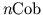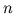Next: Acknowledgements Up: Planck Previous: Higher-Dimensional Algebra

# 6. 4-Dimensional Quantum Gravity

How important are the lessons of topological quantum field theory for 4-dimensional quantum gravity? This is still an open question. Since TQFTs lack local degrees of freedom, they are at best a warmup for the problem we really want to tackle: constructing a background-free quantum theory with local degrees of freedom propagating causally. Thus, even though work on TQFTs has suggested new ideas linking quantum theory and general relativity, these ideas may be too simplistic to be useful in real-world physics.

However, physics is not done by sitting on ones hands and pessimistically pondering the immense magnitude of the problems. For decades our only insights into quantum gravity came from general relativity and quantum field theory on spacetime with a fixed background metric. Now we can view it from a third angle, that of topological quantum field theory. Surely it makes sense to invest some effort in trying to combine the best aspects of all three theories!

And indeed, in the last few years various people have begun to do just this, largely motivated by tantalizing connections between topological quantum field theory and loop quantum gravity. In loop quantum gravity, the preliminary Hilbert space has a basis given by spin networks' -- roughly speaking, graphs with edges labelled by spins [4,29]. We now understand quite well how a spin network describes a quantum state of the geometry of space. But spin networks are also used to describe states in TQFTs, where they arise naturally from considerations of higher-dimensional algebra. For example, in 3-dimensional quantum gravity the state shown in Figure 4 can also be described using the spin network shown in Fig. 6.Using the relationships between 4-dimensional quantum gravity and topological quantum field theory, researchers have begun to formulate theories in which the quantum geometry of spacetime is described using spin foams' -- roughly speaking, 2-dimensional structures made of polygons joined at their edges, with all the polygons being labelled by spins [6,11,16,23,24]. The most important part of a spin foam model is a recipe assigning an amplitude to each spin foam. Much as Feynman diagrams in ordinary quantum field theory describe processes by which one collection of particles evolves into another, spin foams describe processes by which one spin network evolves into another. Indeed, there is a category whose objects are spin networks and whose morphisms are spin foams! And like, this category appears to arise very naturally from purely-categorical considerations.

In the most radical approaches, the concepts of space' and state' are completely merged in the notion of spin network', and similarly the concepts of spacetime' and process' are merged in the notion of spin foam', eliminating the scaffolding of a spacetime manifold entirely. To me, at least, this is a very appealing vision. However, there are a great many obstacles to overcome before we have a full-fledged theory of quantum gravity along these lines. Let me mention just a few of the most pressing. First there is the problem of developing quantum theories of Lorentzian rather than Riemannian metrics. Second, and closely related, we need to better understand the concept of `causal structure' in the context of spin foam models. Only the work of Markopoulou and Smolin  has addressed this point so far. Third, there is the problem of formulating physical questions in these theories in such a way that divergent sums are eliminated. And fourth, there is the problem of developing computational techniques to the point where we can check whether these theories approximate general relativity in the limit of large distance scales -- i.e., distances much greater than the Planck length. Starting from familiar territory we have sailed into strange new waters, but only if we circle back to the physics we know will the journey be complete.

Next: Acknowledgements Up: Planck Previous: Higher-Dimensional Algebra